Chemical equilbrium 2012 VCE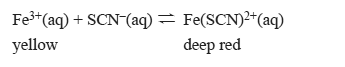1) The concentration profile below represents a change to the above equilibrium system at time t1.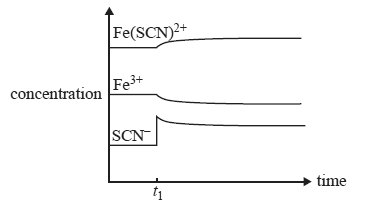a) Which one of the following would account for the changes in concentration at time t1? a) the addition of SCN– b) the removal of Fe(SCN)2+ c) an increase in temperature d) a decrease in temperature Solution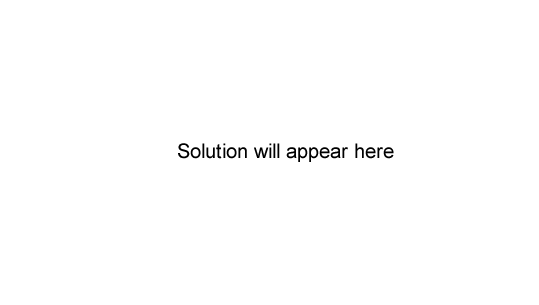b) Which one of the following best represents the changes in concentration when the equilibrium mixture is diluted at time t2?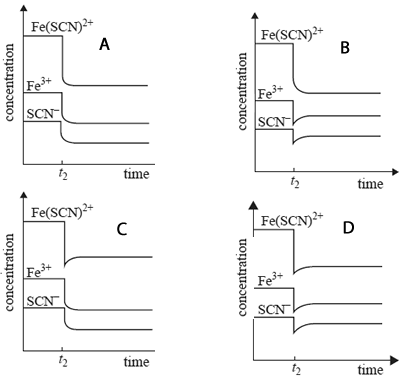Solution 2) The reaction below is used in some industries to produce hydrogen gas.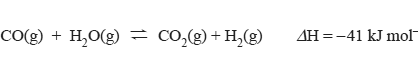a) Carbon monoxide, water vapour, carbon dioxide and hydrogen were pumped into a sealed container that was maintained at a constant temperature of 200 °C. After 30 seconds, the concentration of gases in the sealed container was found to be [CO] = 0.1 M, [H2O] = 0.1 M, [H2] = 2.0 M, [CO2] = 2.0 M. The equilibrium constant at 200 °C for the above reaction is K = 210. Which one of the following statements about the relative rates of the forward reaction and the reverse reaction at 30 seconds is true? a) The rate of the forward reaction is greater than the rate of the reverse reaction. b) The rate of the forward reaction is equal to the rate of the reverse reaction. c) The rate of the forward reaction is less than the rate of the reverse reaction. d) There is insuffi cient information to allow a statement to be made about the relative rates of the forward and reverse reactions. Solutionb) The reaction between carbon monoxide and water vapour is carried out in a sealed container. The equilibrium yield of hydrogen will be increased by a) an increase in pressure at constant temperature. b) a decrease in temperature. c) the addition of an inert gas at constant temperature. d) the use of a suitable catalyst at constant temperature. Solution5) In trials, the reaction above is carried out with and without a catalyst in the sealed container. All other conditions are unchanged. The change in hydrogen concentration with time between an uncatalysed and a catalysed reaction is represented by a graph. Which graph below is correct?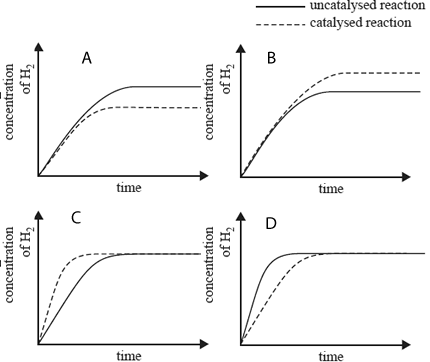Solution6) In an experiment, 1.0 mol of pure phosgene, COCl2, is placed in a 3.0 L fl ask where the following reaction takes place. COCl2(g) <=> CO(g) + Cl2(g) K = 2.1 × 10–8 M a) It can be assumed that, at equilibrium, the amount of unreacted COCl2 is approximately equal to 1.0 mol. On the basis of the data provided, explain why this assumption is justifi ed.   Solutionb) Calculate the equilibrium concentration, in mol L–1, of carbon monoxide, CO. Assume that the amount of unreacted COCl2 is approximately equal to 1.0 mol.   Solutionc) What is the equilibrium concentration of chlorine gas? Solution 7) Carbon monoxide, water vapour, carbon dioxide and hydrogen were pumped into a sealed container and allowed to reach equilibrium according to the equatin below.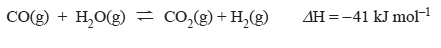a) The mixture was maintained at a constant temperature of 200 °C. After 30 seconds, the concentration of gases in the sealed container was found to be [CO] = 0.1 M, [H2O] = 0.1 M, [H2] = 2.0 M, [CO2] = 2.0 M. The equilibrium constant at 200 °C for the above reaction is K = 210. Which one of the following statements about the relative rates of the forward reaction and the reverse reaction at 30 seconds is true? A. The rate of the forward reaction is greater than the rate of the reverse reaction. B. The rate of the forward reaction is equal to the rate of the reverse reaction. C. The rate of the forward reaction is less than the rate of the reverse reaction. D. There is insuffi cient information to allow a statement to be made about the relative rates of the forward and reverse reactions. Solutionb) The reaction between carbon monoxide and water vapour is carried out in a sealed container. The equilibrium yield of hydrogen will be increased by A. an increase in pressure at constant temperature. B. a decrease in temperature. C. the addition of an inert gas at constant temperature. D. the use of a suitable catalyst at constant temperature. Solution